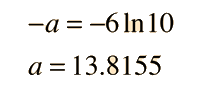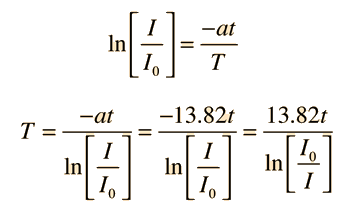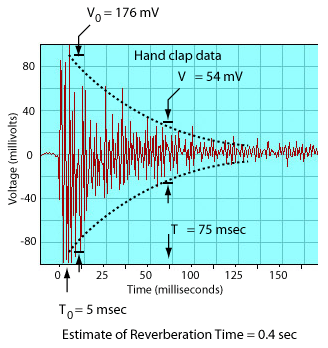# Reverberation Time Estimate

One way to estimate the reverberation time of a room is to make a plot of the decay pattern from a sharp sound and approximate it with an exponential. The standard reverberation time is the time to drop 60 decibels, or to one-millionth or 10-6 of the original level. If that time can be projected from the exponential decay, we have an estimate of the reverberation time of the room.Taking the natural log of both sides gives:Having evaluated the constant a, the expressions can be rearranged to give an expression for the reverberation time T:If a voltage proportional to the sound amplitude is being measured with a microphone, then in terms of the measured voltages the expression for reverberation time T is:The last step makes use of the fact that the sound intensity is proportional to the square of the amplitude of the wave, and that most microphones produce an output voltage which is proportional to the amplitude of the pressure wave. Since ln(x2) = 2ln(x), the constant a is divided by 2.

If the amplitude drops from V0 = volts to V = volts ,

a drop to x its original value,

in time t = seconds,

then the Reverberation Time T = seconds.Above is an example of data from a handclap. There are lots of "real world" problems with this approach, and the only thing that can really be claimed is that it gives a real impression of the nature of sound decay in a room. The decay envelope is not symmetric about the horizontal axis, it is not a smooth decay, etc. The equipment used was just a microphone connected to a laptop computer and the software MacScope II from Elisha Huggins which gave an oscilloscope-type display of the microphone output as a function of time.

 Discussion Calculation
Index

Auditorium acoustics

 HyperPhysics***** Sound R Nave
Go Back